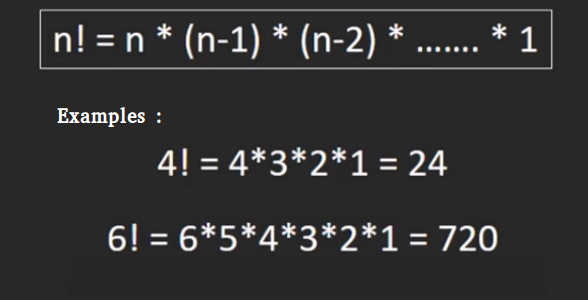# Java Program for factorial of a number

Factorial of a non-negative integer, is multiplication of all integers smaller than or equal to n. For example factorial of 6 is 6*5*4*3*2*1 which is 720.Recursive :

 `// Java program to find factorial of given number ` `class` `Test ` `{ ` `    ``// method to find factorial of given number ` `    ``static` `int` `factorial(``int` `n) ` `    ``{ ` `        ``if` `(n == ``0``) ` `          ``return` `1``; ` `         `  `        ``return` `n*factorial(n-``1``); ` `    ``} ` `     `  `    ``// Driver method ` `    ``public` `static` `void` `main(String[] args)  ` `    ``{ ` `        ``int` `num = ``5``; ` `        ``System.out.println(``"Factorial of "``+ num + ``" is "` `+ factorial(``5``)); ` `    ``} ` `} `

Iterative Solution:

 `// Java program to find factorial of given number ` `class` `Test ` `{ ` `    ``// Method to find factorial of given number ` `    ``static` `int` `factorial(``int` `n) ` `    ``{ ` `        ``int` `res = ``1``, i; ` `        ``for` `(i=``2``; i<=n; i++) ` `            ``res *= i; ` `        ``return` `res; ` `    ``} ` `     `  `    ``// Driver method ` `    ``public` `static` `void` `main(String[] args)  ` `    ``{ ` `        ``int` `num = ``5``; ` `        ``System.out.println(``"Factorial of "``+ num + ``" is "` `+ factorial(``5``)); ` `    ``} ` `} `

One line Solution (Using Ternary operator):

 `// Java program to find factorial ` `// of given number ` `class` `Factorial { ` ` `  `  ``int` `factorial(``int` `n) ` `  ``{ ` ` `  `    ``// single line to find factorial ` `    ``return` `(n == ``1` `|| n == ``0``) ? ``1` `: n * factorial(n - ``1``); ` ` `  `  ``} ` ` `  ` `  `  ``// Driver Code ` `  ``public` `static` `void` `main(String args[])      ` `  ``{ ` `    ``Factorial obj = ``new` `Factorial(); ` `    ``int` `num = ``5``; ` `    ``System.out.println(``"Factorial of "` `+ num +  ` `                      ``" is "` `+ obj.factorial(num)); ` `   ``} ` `} ` `// This code is contributed by Anshika Goyal. `

The above solutions cause overflow for small numbers. Please refer factorial of large number for a solution that works for large numbers.

Please refer complete article on Program for factorial of a number for more details!

My Personal Notes arrow_drop_up
Article Tags :
Practice Tags :

4

Please write to us at contribute@geeksforgeeks.org to report any issue with the above content.